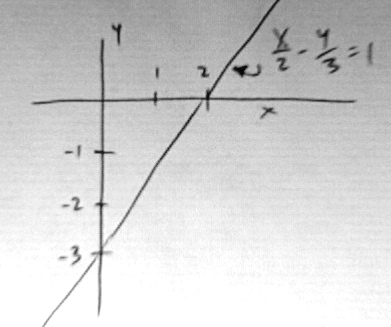# How Do You Graph A Linear Equation?

The picture provides an illustration. The equation in the picture is in a particular form called "intercept form." In this form, the equation is (x/x-intercept) + (y/y-intercept) = 1. You can see that when x=0, the equation is satisfied by y = y-intercept. Likewise, when y=0, the equation is satisfied by x = x-intercept. This makes graphing the equation pretty easy, as all you must do is locate a point on the x-axis (the x-intercept), and a point on the y-axis (the y-intercept). The line goes through those points.